Take the Last leap| Aakash BYJU'S JEE (Adv) '23 Mock Test on 28th May Take the Last leap| Aakash BYJU'S JEE (Adv) '23 Mock Test on 28th May

# JEE Main 2020 Maths Paper With Solutions Shift 2 Sept 4

JEE Main 2020 Maths paper September 4 Shift 2 solutions are available on this page. These are solved by subject experts at BYJU’S. Practising previous question papers is one of the best methods for JEE preparation. Learning these problems will help students to get an idea of the exam pattern of the JEE Main exam. Aspirants can easily download the PDF format of these solutions for free.

## JEE Main 2020 Maths Paper With Solutions Shift 2 September 4

September 4 Shift 2 – Maths

1. Suppose the vectors x1, x2 and x3 are the solutions of the system of linear equations, Ax = b when the vector b on the right side is equal to b1, b2 and b3 respectively. If x1 =

$$\begin{array}{l}\begin{bmatrix} 1\\ 1\\ 1 \end{bmatrix}\end{array}$$
, x2 =
$$\begin{array}{l}\begin{bmatrix} 0\\ 2\\ 1 \end{bmatrix}\end{array}$$
, x3 =
$$\begin{array}{l}\begin{bmatrix} 0\\ 0\\ 1 \end{bmatrix}\end{array}$$
, b1=
$$\begin{array}{l}\begin{bmatrix} 1\\ 0\\ 0 \end{bmatrix}\end{array}$$
, b2 =
$$\begin{array}{l}\begin{bmatrix} 0\\ 2\\ 0 \end{bmatrix}\end{array}$$
, b3 =
$$\begin{array}{l}\begin{bmatrix} 0\\ 2\\ 2 \end{bmatrix}\end{array}$$
, then the determinant of A is equal to

a) 2
b) 1/2
c) 3/2
d) 4

Using AX = B

A =

$$\begin{array}{l}\begin{bmatrix} a_{1} & a_{2} &a_{3} \\ a_{4}& a_{5} & a_{6} \\ a_{7}& a_{8} & a_{9} \end{bmatrix}\end{array}$$

a1+a2+a3 = 1

a4+a5+a6 = 0

a7+a8+a9 = 0

2a2+a3 = 0

2a5+a6 = 2

2a8+a9 = 0

a3 = 0, a6 = 0, a9 = 2

a8 = -1

a5 = 1

a2 = 0

⇒ a1 = 1

a4 = -1

a7 = -1

A =

$$\begin{array}{l}\begin{bmatrix} 1 & 0 & 0\\ -1& 1 & 0\\ -1& -1 & 2 \end{bmatrix}\end{array}$$

A = 2×1 = 2

2. If a and b are real numbers such that (2+α)4 = a+bα, where α = (-1+i√3)/2 then a+b is equal to:

a) 33
b) 57
c) 9
d) 24

(2+α)4 = a+bα

(2+(-1+i√3)/2)4 = a+bα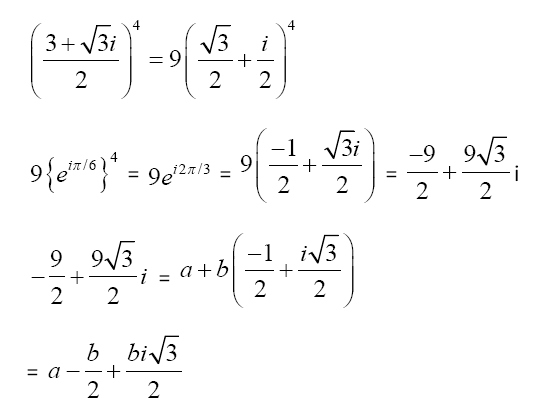b√3/2 = 9√3/2

⇒ b = 9

a = 0

a+b = 9

3. The distance of the point (1, -2, 3) from the plane x-y+z = 5 measured parallel to the line (x/2) = (y/3) = (z/-6) is:

a) 1/7
b) 7
c) 7/5
d) 1

Equation of line through (1,-2,3) whose d.r.s. are (2,3,-6)

(x-1)/2 = (y+2)/3 = (z-3)/-6 = λ

Any point on the line (2λ+1, 3λ-2, -6λ+3)

Put in (x-y+z = 5)

2λ+1- 3λ+2-6λ+3 = 5

-7λ = -1

λ = 1/7

Distance = √((2λ)2+(3λ)2+(6λ)2)

= √(4λ2+9λ2+36λ2)

= 7λ

= 1 unit

4. Let f: (0, ∞)→ (0, ∞) be a differentiable function such that f(1) = e and

$$\begin{array}{l}\lim_{t\to x}\frac{t^{2}f^{2}(x)-x^{2}f^{2}(t)}{t-x}=0\end{array}$$
. If f(x) = 1, then x is equal to:

a) e
b) 2e
c) 1/e
d) 1/2e

f(1) = e ..(i)

$$\begin{array}{l}\lim_{x\to0}\frac{t^{2}f^{2}(x)-x^{2}f^{2}(t)}{t-x}=0\end{array}$$

L’ Hospital rule

⇒ limt→x (2tf2(x)-2x2f(t).f’(t)) = 0

⇒ 2xf2(x)-2x2f(x).f’(x) = 0

⇒ 2xf(x){f(x)-xf’(x)} = 0

⇒ f’(x)/f(x) = 1/x

ln f(x) = ln x+ln c

⇒ f(x) = cx ..(ii)

If x = 1

f(1) = c(1)

f(1) = c

From equation (i) and (ii)

c = e ..(iii)

From (iii)

f(x) = ex

⇒ y = ex or y = cx

if f(x) = 1

⇒ x = 1/e

5. Contrapositive of the statement :

‘If a function f is differentiable at a, then it is also continuous at a’, is:

a) If a function f is not continuous at a, then it is not differentiable at a.
b) If a function f is continuous at a, then it is differentiable at a.
c) If a function f is continuous at a, then it is not differentiable at a.
d) If a function f is not continuous at a, then it is differentiable at a.

Contrapositive of p → q = ∼q → ∼p

6. The minimum value of 2sinx+2cosx is:

a) 21-√2
b) 21-1/√2
c) 2-1+√2
d) 2-1+1/√2

Using A.M. ≥ G.M.

y = 2sinx+2cosx

(2sinx+2cosx)/2 ≥ √(2sinx+cosx)

2sinx+cosx ≥ 21×2(sinx+cosx)/2

2sinx+cosx ≥ 2(2+sinx+cosx)/2

⇒ (2sin x+2cos x)minimum = 2(2-√2)/2

= 21-1/√2

7. If the perpendicular bisector of the line segment joining the points P(1 ,4) and Q(k, 3) has y-intercept equal to -4, then a value of k is:

a) -2
b) √15
c) √14
d) -4

mPQ = (4-3)/(1-k)

$$\begin{array}{l}m_{\perp}\end{array}$$
= k-1

Midpoint of PQ = ((k+1)/2, 7/2)

equation of perpendicular bisector

y-7/2 = (k-1)(x-(k+1)/2)

for y intercept put x = 0

y = (7/2)-(k2-1)/2 = -4

(k2-1)/2 = 15/2

⇒ k = 4 and k = -4

8. The area (in sq. units) of the largest rectangle ABCD whose vertices A and B lie on the x-axis and vertices C and D lie on the parabola, y = x2-1 below the x-axis, is:

a) 2/3√3
b) 4/3
c) 1/3√3
d) 4/3√3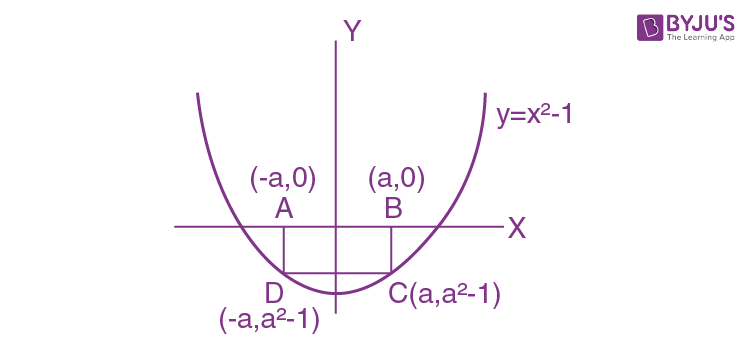Area = 2a(a2-1)

A = 2a3-2a

dA/da = 6a2-2 = 0

d2A/da2 = 12a

at a = -1/√3

d2A/da2 = -4√3 <0

So area is maximum at a = -1/√3

Amax = (-2/3√3)+(2/√3)

= (-2+6)/3√3

= 4/3√3 sq. units

9. The integral

$$\begin{array}{l}\int_{\frac{\pi }{6}}^{\frac{\pi }{3}}\tan ^{3}x.\sin ^{2}3x(2\sec ^{2}x.\sin ^{2}3x+3\tan x.\sin 6x)dx\end{array}$$
is equal to

a) 9/2
b) -1/18
c) -1/9
d) 7/18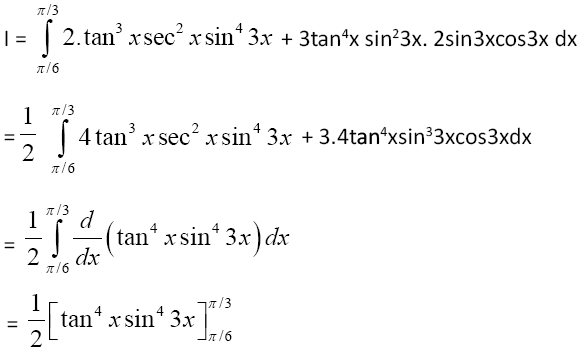= (1/2)[9×0-(1/3)×(1/3)×1]

= -1/18

10. If the system of equations

x+y+z = 2

2x+4y–z = 6

3x+2y+ λz = μ

has infinitely many solutions, then

a) λ-2μ = -5
b) 2λ+μ = 14
c) λ+2μ = 14
d) 2λ-μ = 5

D = 0

$$\begin{array}{l}\begin{vmatrix} 1 & 1 & 1\\ 2& 4 & -1\\ 3 & 2 & \lambda \end{vmatrix}\end{array}$$
= 0

(4λ+2)-1(2λ+3)+1(4-12) = 0

4λ+2-2λ-3-8 = 0

2λ = 9

⇒ λ = 9/2

Dx =

$$\begin{array}{l}\begin{vmatrix} 2 & 1 & 1\\ 6& 4 & -1\\ \mu & 2 & \frac{9}{2} \end{vmatrix}\end{array}$$
= 0

⇒ μ = 5

Now check option

2λ+μ = 14

11. In a game two players A and B take turns in throwing a pair of fair dice starting with player A and total of scores on the two dice, in each throw is noted. A wins the game if he throws a total of 6 before B throws a total of 7 and B wins the game if he throws a total of 7 before A throws a total of six The game stops as soon as either of the players wins. The probability of A winning the game is :

a) 5/31
b) 31/61
c) 30/61
d) 5/6

sum total 7 = {(1,6)(2,5)(3,4)(4,3)(5,2)(6,1)}

P(sum 7) = 6/36

sum total 6 = {(1,5)(2,4)(3,3)(4,2)(5,1)}

P(sum 6) = 5/36

P(Awin) =

$$\begin{array}{l}P(6)+P(\bar{6}).P(\bar{7}).P(6)+..\end{array}$$

= (5/36)+(31/36)×(30/36)×(5/36)+…

= (5/36)÷(1-(31×30)/(36×36)

= (5×36)/(36×36-31×30)

= 5×36/366

= 30/61

12. If for some positive integer n, the coefficients of three consecutive terms in the binomial expansion of (1+x)n+5 are in the ratio 5:10:14, then the largest coefficient in this expansion is :

a) 792
b) 252
c) 462
d) 330

Tr:Tr+1:Tr+2

n+5Cr-1: n+5Cr: n+5Cr+1 = 5:10:14

(n+5)!/(r-1)!(n+6-r)! : (n+5)!/r!(n+5-r)! = 5/10

r/(n+6-r) = 1/2

(r+1)!(n+4-r)!/r!(n+5-r)! = 5/7

2r = n+6-r

3r = n+6 ..(i)

(r+1)/(n+5-r) = 5/7

7r+7 = 5n + 25-5r

12r = 5n+18 ..(ii)

From eq.(i) and (ii)

4(n+6) = 5n+18

n = 6, r = 4

Then we can find (1+x)11

Largest coefficient in the expansion (1+x)11 = 11C6 = 462

13. The function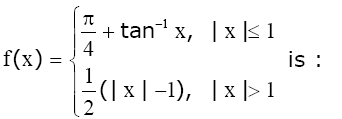a) both continuous and differentiable on R-{-1}
b) continuous on R-{-1} and differentiable on R-{-1,1}
c) continuous on R-{1} and differentiable on R-{-1,1}
d) both continuous and differentiable on R-{1}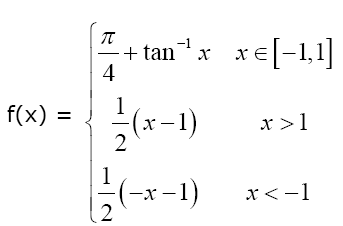At x = 1, f(1) = π/2

f(1+) = 0

discontinuous ⇒ non diff.

At x = -1

f(-1) = 0

f(-1) = (1/2){+1-1} = 0

cont. at x = -1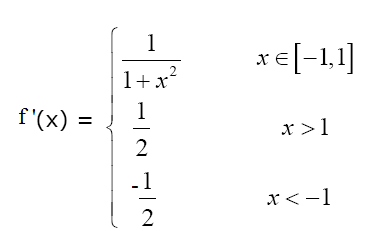At x = -1, f’(-1) = -1/2

And f’(-1+) = 1/2

So, at x = -1 it is non differentiable.

14. The solution of the differential equation (dy/dx)-((y+3x)/loge(y+3x)) + 3 = 0 is: (where c is a constant of integration)

a) x-loge(y+3x) = C
b) x-(1/2)(loge(y+3x))2 = C
c) x-2loge(y+3x) = C
d) y+3x-(1/2)(logex)2 = C

(dy/dx)-((y+3x)/ln(y+3x))+3 = 0

⇒ (dy/dx)+3 = (y+3x)/ln(y+3x)

Let ln(y + 3x) = t

(1/y+3x)×((dy/dx)+3) = dt/dx

(y+3x)dt/dx = (y+3x)/t

⇒ tdt = dx

t2/2 = x+C

(1/2)(ln(y+3x))2 = x+C

15. Let λ ≠ 0 be in R. If α and β are the roots of the equation, x2-x+2λ = 0 and α and γ are the roots of the equation, 3x2-10x+27λ = 0, then βγ/λ is equal to:

a) 27
b) 9
c) 18
d) 36

x2-x+2λ = 0 (α, β) ..(i)

3x2-10x+27λ = 0 (α, γ) ..(ii)

Multiply (i) by 3

3x2-3x+6λ = 0 ..(iii)

Equation (ii)-(iii)

-7x+21λ = 0

α = 3λ

Put in equation (i)

2-3λ+2λ = 0

2-λ = 0

⇒ λ = 1/9

⇒ α = 1/3

αβ = 2/9

⇒ β = 2/3

αγ = 1

⇒ γ = 3

βγ/λ ⇒ (2/3)×3÷1/9

= 18

16. The angle of elevation of a cloud C from a point P, 200 m above a still lake is 300. If the angle of depression of the image of C in the lake from the point P is 600, then PC (in m) is equal to :

a) 200√3
b) 400√3
c) 400
d) 100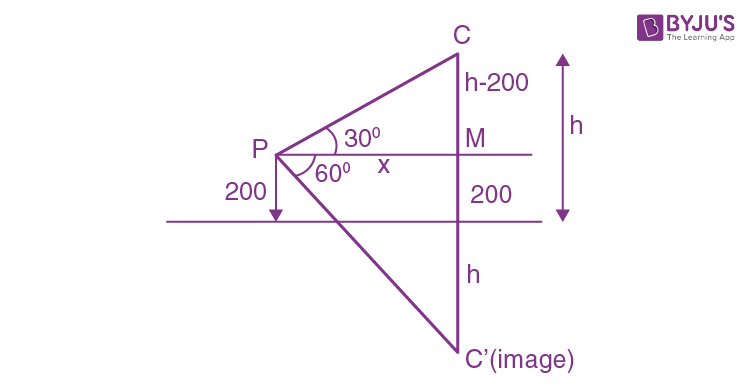(h-200)/x = tan 300

(h+200)/x = tan 600

(h+200)/(h-200) = 3

h+200 = 3h-600

2h = 800

h = 400

(h-200)/PC = sin 300

PC = 400 m

17. Let

$$\begin{array}{l}\bigcup_{i=1}^{50}X_{i}= \bigcup_{i=1}^{n}Y_{i}= T\end{array}$$
where each Xi contains 10 elements and each Yi contains 5 elements. If each element of the set T is an element of exactly 20 of sets Xi ’s and exactly 6 of sets Yi’s, then n is equal to :

a) 15
b) 30
c) 50
d) 45

Number of elements of set T =

$$\begin{array}{l}\bigcup_{i=1}^{50}X_{i}= \bigcup_{i=1}^{n}Y_{i}\end{array}$$

⇒ 50×10/20 = n×5/6

⇒ (50/2)×(6/5) = n

⇒ n = 30

18. Let x = 4 be a directrix to an ellipse whose centre is at the origin and its eccentricity is 1/2. If P(1, β ), β> 0 is a point on this ellipse, then the equation of the normal to it at P is :

a) 8x-2y = 5
b) 4x-2y = 1
c) 7x-4y = 1
d) 4x-3y = 2

e = 1/2

x = a/e = 4

⇒ a = 2

e2 = 1-b2/a2

⇒ 1/4 = 1-(b2/4)

(b2/4) = 3/4

⇒ b2 = 3

Elipse (x2/4)+(y2/3) =1

P(1,β)

x = 1

(1/4)+(β2/3) = 1

β2/3 = 3/4

⇒ β = 3/2

⇒ P(1, 3/2)

Equation of normal (a2x/x1)-(b2y/y1) = a2-b2

(4x/1)-(3y/3/2) = 4-3

4x-2y = 1

19. Let a1, a2, …, an be a given A.P. whose common difference is an integer and Sn = a1+a2+ …. +an. If a1 = 1, an = 300 and 15 ≤ n ≤ 50, then the ordered pair (Sn-4, an-4) is equal to:

a) (2480,248)
b) (2480,249)
c) (2490,249)
d) (2490,248)

a1 = 1, an = 300, 15≤ n ≤50

300 = 1+(n-1)d

(n-1) = 299/d

n-1 = integer, d has to be a factor of 299.

So, d = 23 or 13

if d = 23

then n-1 = 13

n = 14 (reject)

or d = 13

n-1 = 23

n = 24 is possible (15 ≤ n <50)

Or S20 = (20/2){2+19×13}

= 10×249

= 2490

a20 = 1+19×13

= 248

(S20, a20) = (2490, 248)

20. The circle passing through the intersection of the circles, x2+y2-6x = 0 and x2+y2-4y = 0, having its centre on the line, 2x-3y+12 = 0, also passes through the point:

a) (-1,3)
b) (1,-3)
c) (-3,6)
d) (-3,1)

S1+λ(S1-S2) = 0

x2 + y2-6x+λ(4y-6x) = 0

x2+y2-6x(1+ λ)+4λy = 0

Centre (3(1+λ ), -2λ) put in 2x-3y+12 = 0

6+6λ+6λ+12 = 0

12λ = -18

λ = -3/2

Circle is x2+y2+3x-6y = 0

Check options

(-3,6) is the point.

21. Let {x} and [x] denote the fractional part of x and the greatest integer ≤ x respectively of a real number x. If

$$\begin{array}{l}\int_{0}^{n}{x}dx\end{array}$$
,
$$\begin{array}{l}\int_{0}^{n}[x]dx\end{array}$$
and 10(n2-n), (nN, n>1) are three consecutive terms of a G.P., then n is equal to

$$\begin{array}{l}\int_{0}^{n}\left \{ x \right \}dx = n\int_{0}^{1}x dx\end{array}$$

=

$$\begin{array}{l}n\left ( \frac{x^{2}}{2}\right )_{0}^{1}\end{array}$$

= n/2

$$\begin{array}{l}\int_{0}^{n}[x ]dx = \int_{0}^{1}0dx+ \int_{1}^{2}1dx +\int_{2}^{3}2dx+..\int_{n-1}^{n}(n-1)dx\end{array}$$

⇒ 1+2+…n-1 = n(n-1)/2

⇒ n/2, n(n-1)/2, 10(n2-n) → G.P

⇒ n2(n-1)2/4 = (n/2)×10×n×(n-1)

⇒ n-1 = 20

⇒ n = 21

22. A test consists of 6 multiple choice questions, each having 4 alternative answers of which only one is correct. The number of ways, in which a candidate answers all six questions such that exactly four of the answers are correct, is

6C4×1×32 = 15×9 = 135

23. If

$$\begin{array}{l}\vec{a}= 2\hat{i}+\hat{j}+2\hat{k}\end{array}$$
, then the value of
$$\begin{array}{l}\left | \hat{i} \times (\vec{a}\times \hat{i})\right |^{2}+\left | \hat{j} \times (\vec{a}\times \hat{j})\right |^{2}+\left | \hat{k} \times (\vec{a}\times \hat{k})\right |^{2}\end{array}$$
is equal to:

$$\begin{array}{l}\left | \hat{i} \times (\vec{a}\times \hat{i})\right |^{2} = \left | \vec{a} -(a.\hat{i})\hat{i}\right |^{2}\end{array}$$

=

$$\begin{array}{l}\left | \hat{j}+2\hat{k} \right |^{2}\end{array}$$

= 1+4

= 5

Similarly

$$\begin{array}{l}\left | \hat{j} \times (\vec{a}\times \hat{j})\right |^{2} =\left | 2\hat{i}+2\hat{k} \right |^{2}\end{array}$$
= 4+4

= 8

$$\begin{array}{l}\left | \hat{k} \times (\vec{a}\times \hat{k})\right |^{2} =\left | 2\hat{i}+\hat{j} \right |^{2}\end{array}$$
= 4+1

= 5

⇒ 5+8+5 = 18

24. Let PQ be a diameter of the circle x2+y2 = 9. If α and β are the lengths of the perpendiculars from P and Q on the straight line, x+y = 2 respectively, then the maximum value of αβ is: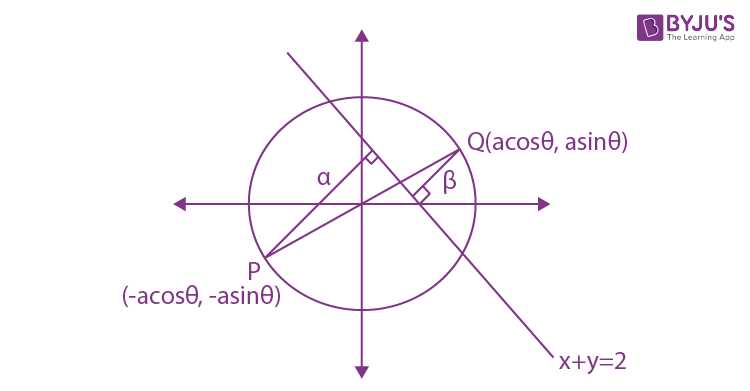α =

$$\begin{array}{l}\left | \frac{3\cos \theta +3\sin \theta -2}{\sqrt{2}} \right |\end{array}$$

β =

$$\begin{array}{l}\left | \frac{+3\cos \theta +3\sin \theta +2}{\sqrt{2}} \right |\end{array}$$

αβ =

$$\begin{array}{l}\left | \frac{(3\cos \theta +3\sin \theta)^{2} -4}{{2}} \right |\end{array}$$

⇒ αβ =

$$\begin{array}{l}\left | \frac{9 +9\sin 2\theta -4}{{2}} \right |\end{array}$$

⇒ αβ =

$$\begin{array}{l}\left | \frac{5 +9\sin 2\theta }{{2}} \right |\end{array}$$

αβmax = (9+5)/2

= 7

25. If the variance of the following frequency distribution :

 Class 10-20 20-30 30-40 Frequency 2 x 2

is 50, then x is equal to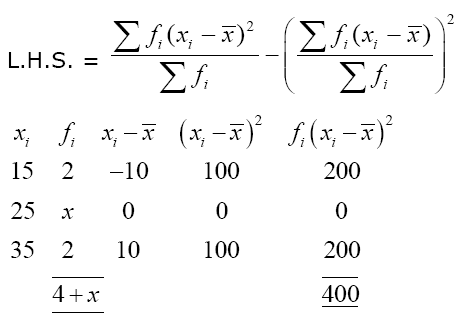$$\begin{array}{l}\bar{x}\end{array}$$
= (100+25x)/(4+x)

$$\begin{array}{l}\bar{x}\end{array}$$
= 25

400/(4+x) = 50

x = 4